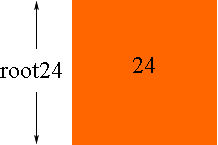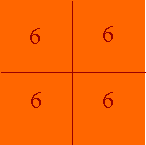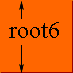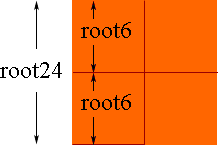Quandaries and Queries u Hi, I know that all algerbra can be represented visually, for example: Y=9x+3 is a line on a two dimentional plane. Could Math Central please send me a visual representation of the proccess of simplifying square root like root24 equals 2root6 Thank you, Burke (Grade 11 student) Hi Burke, Draw a square that has area 24 square units then the length a side of the square is root24 units.Now divide the square into 4. Each piece then has area 6 square units.But a square of area 6 square units has side length of root6 unitsThus root24 = 2 root6Penny Go to Math Central